Anzeige

# TOC Introduction.pptx

29. Mar 2023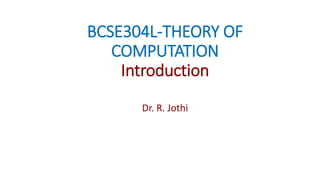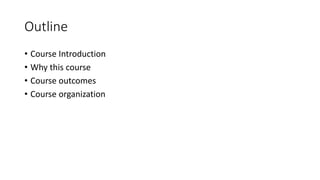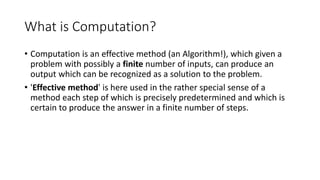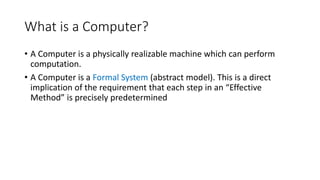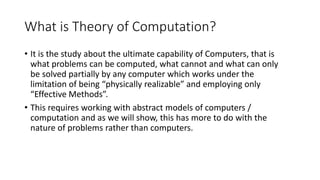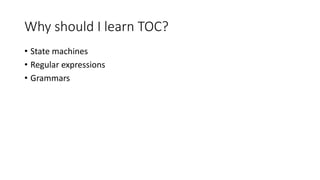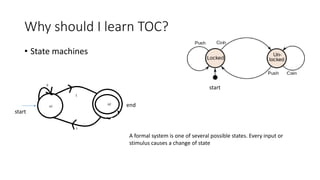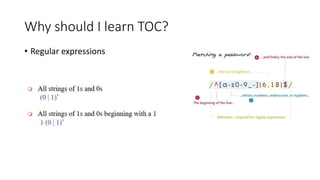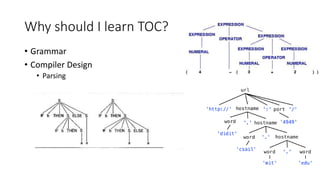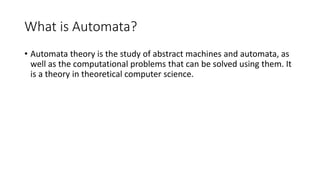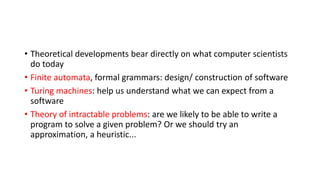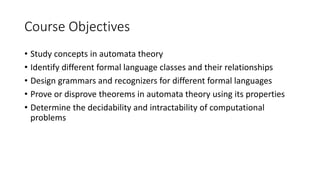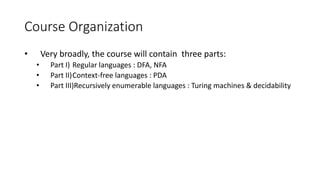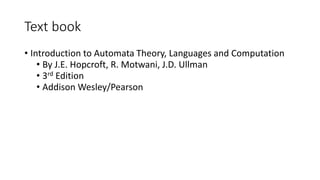1 von 15
Anzeige

### TOC Introduction.pptx

1. BCSE304L-THEORY OF COMPUTATION Introduction Dr. R. Jothi
2. Outline • Course Introduction • Why this course • Course outcomes • Course organization
3. What is Computation? • Computation is an effective method (an Algorithm!), which given a problem with possibly a finite number of inputs, can produce an output which can be recognized as a solution to the problem. • 'Effective method' is here used in the rather special sense of a method each step of which is precisely predetermined and which is certain to produce the answer in a finite number of steps.
4. What is a Computer? • A Computer is a physically realizable machine which can perform computation. • A Computer is a Formal System (abstract model). This is a direct implication of the requirement that each step in an “Effective Method” is precisely predetermined
5. What is Theory of Computation? • It is the study about the ultimate capability of Computers, that is what problems can be computed, what cannot and what can only be solved partially by any computer which works under the limitation of being “physically realizable” and employing only “Effective Methods”. • This requires working with abstract models of computers / computation and as we will show, this has more to do with the nature of problems rather than computers.
6. Why should I learn TOC? • State machines • Regular expressions • Grammars
7. Why should I learn TOC? • State machines start start end A formal system is one of several possible states. Every input or stimulus causes a change of state
8. Why should I learn TOC? • Regular expressions
9. Why should I learn TOC? • Grammar • Compiler Design • Parsing
10. What is Automata? • Automata theory is the study of abstract machines and automata, as well as the computational problems that can be solved using them. It is a theory in theoretical computer science.
11. • Theoretical developments bear directly on what computer scientists do today • Finite automata, formal grammars: design/ construction of software • Turing machines: help us understand what we can expect from a software • Theory of intractable problems: are we likely to be able to write a program to solve a given problem? Or we should try an approximation, a heuristic...
12. Applications • Compiler Design • Natural language processing • Speech recognition • Search engines (finding text pattern in web pages) • Networking protocols • VLSI design
13. Course Objectives • Study concepts in automata theory • Identify different formal language classes and their relationships • Design grammars and recognizers for different formal languages • Prove or disprove theorems in automata theory using its properties • Determine the decidability and intractability of computational problems
14. Course Organization • Very broadly, the course will contain three parts: • Part I) Regular languages : DFA, NFA • Part II)Context-free languages : PDA • Part III)Recursively enumerable languages : Turing machines & decidability
15. Text book • Introduction to Automata Theory, Languages and Computation • By J.E. Hopcroft, R. Motwani, J.D. Ullman • 3rd Edition • Addison Wesley/Pearson

### Hinweis der Redaktion

1. School of EECS, WSU
Anzeige